### Home > INT3 > Chapter 10 > Lesson 10.2.2 > Problem10-125

10-125.
1. 10-125.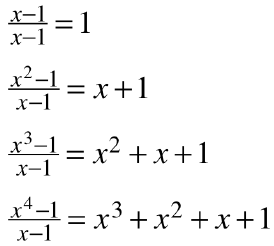Josephina thinks that she has discovered a new pattern, shown at right. Homework Help ✎

1. Check her equations by multiplying. Does her pattern work?

2. Based on her pattern, what does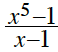equal? Is it true? Justify your answer.

3. Make a conjecture about how to represent the result for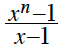. You will need “…” in the middle of your expression.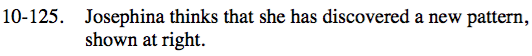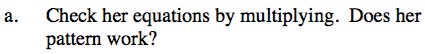To check a division problem using multiplication, multiply the divisor by the quotient. In the second case, multiply (x − 1)(x + 1). Does it equal x2 − 1? How will you check the third and fourth cases?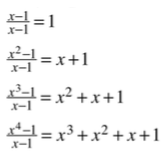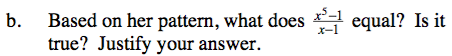Look for a pattern in the quotients. Look at the number of terms and the exponent on each term.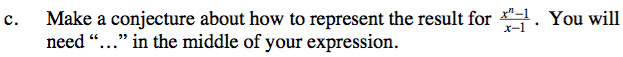$x^n + x^{n-1} + x^{n-2} +...+ x + 1$